Tetration Forum

You're currently viewing a stripped down version of our content. View the full version with proper formatting.
Pages: 1 2 3 4 5 6
James, vacationing; can't add any more calculations for a few days.  The key question seems to be whether the resultant Tetration function converges everywhere  in the complex plane if 0<|Im(z)|<pi, especially near where Beta(z+2)=1, |Beta(z+1)| is small.
After a few days of work (more on fixing bugs in my own drawing program) I got these high value images.

I am now starting to believe that "James' beta method" really shows the truth about "Tetrationess".

The plotted objective function is beta(z,1), and the gray inside the plot indicates Overflow/Underflow

base = e^-e. A very familiar graphic pattern appears here, which is a very good sign!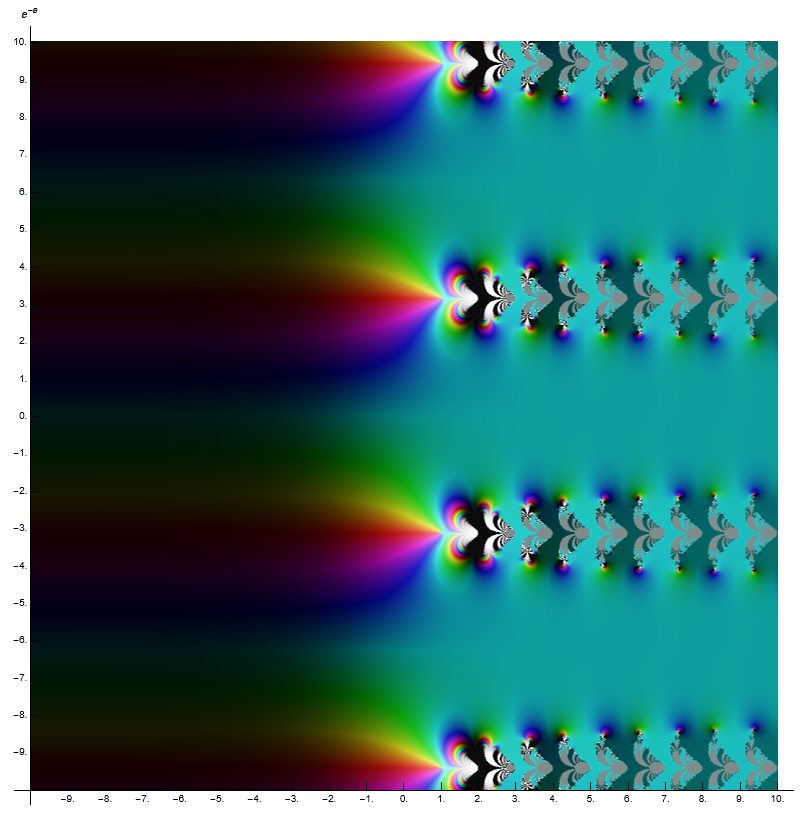base = 10^-5.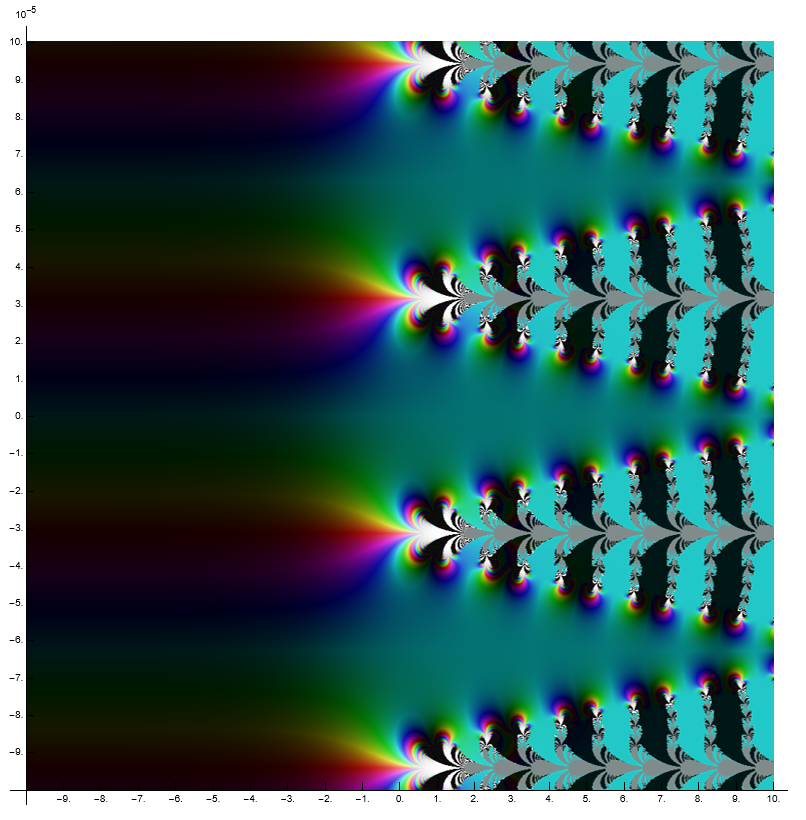You can see the "fusion" between function branches here.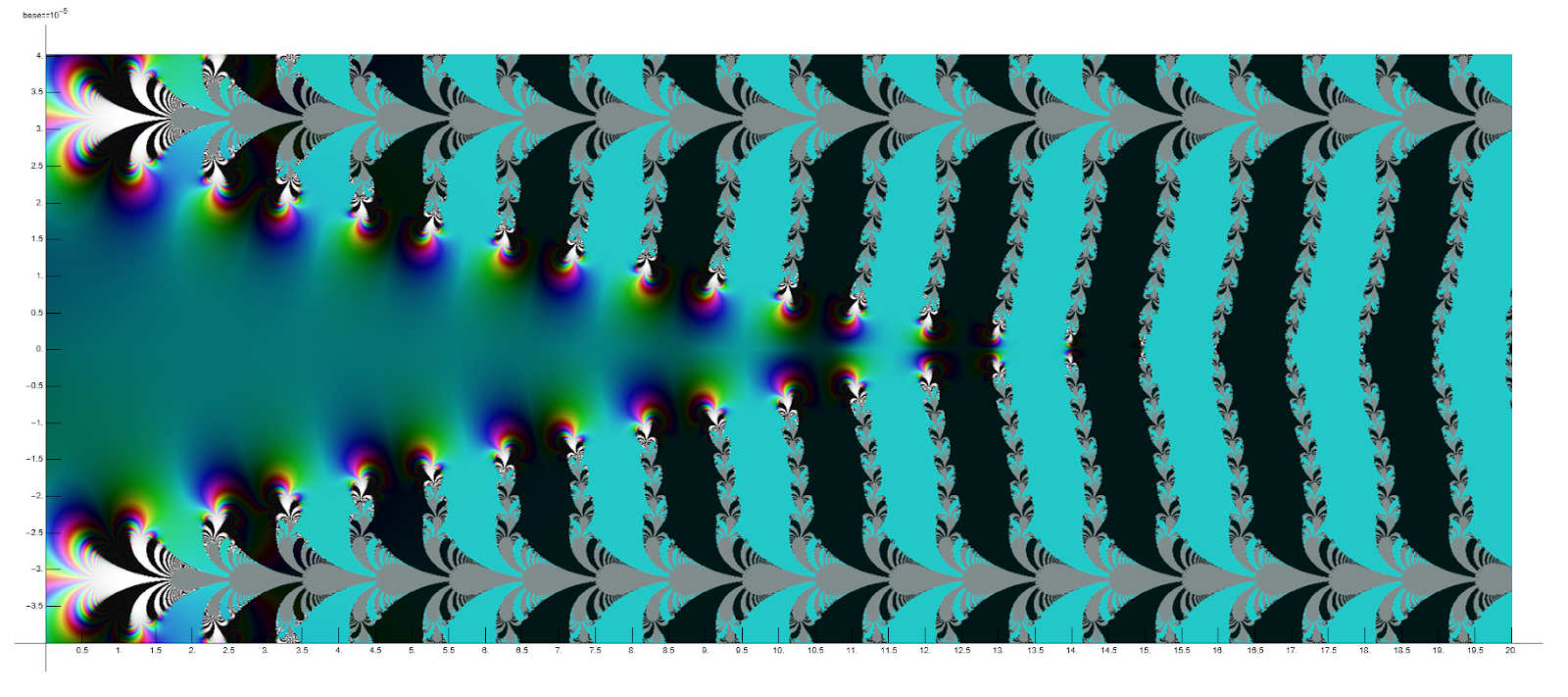base = 10^5. There is an old rumor on the forum that claims that when base -> ∞, there is also some stable Tetrationess behavior.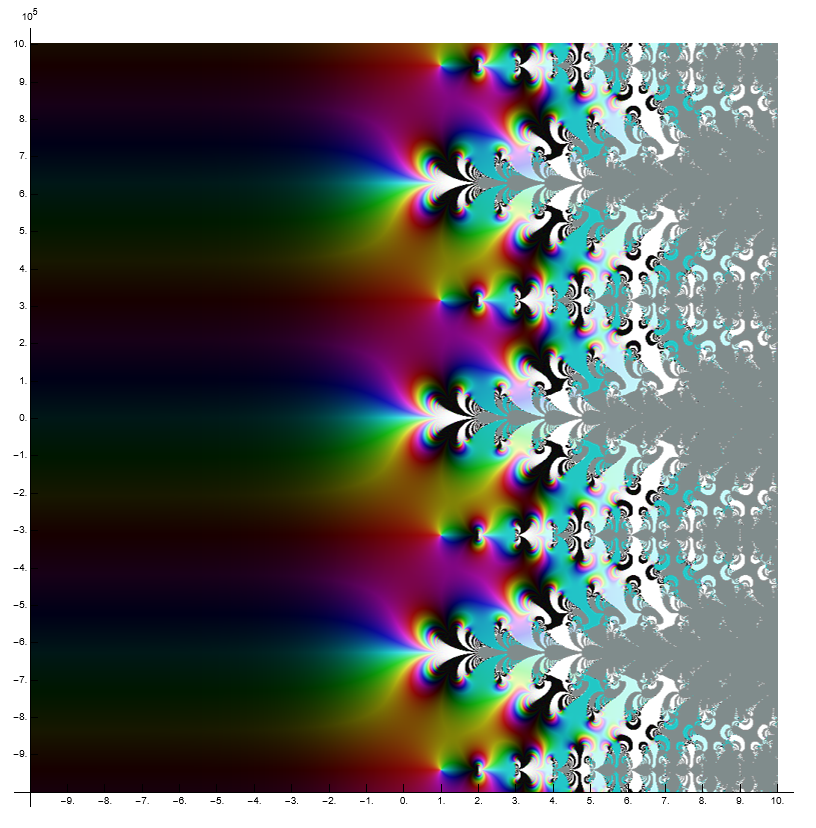base=-99999, Because I was too sleepy I didn't check the product of the input command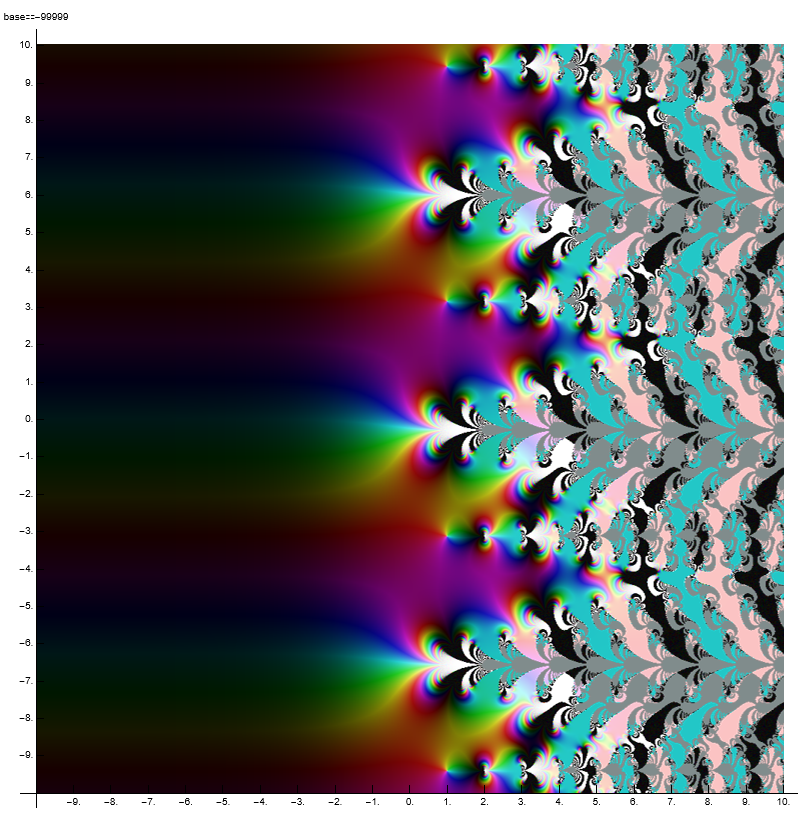You can also see the behavior of the "half-cycle" function similar to base=1E5.

It is worth noting that the base=-1, -1E-5 program cannot calculate

base=1+1E-5, 1-1E-5, 0.5, E^E^-1 nothing special, you can see for yourself in the google drive.
That is absolutely beautiful, Ember! Absolutely beautiful.

The real question is, can we map the strip into the upper half plane.

Very beautiful, Ember. I'll go through the drive soon.

Thanks a lot.

Regards, James
(10/02/2021, 11:36 AM)sheldonison Wrote: [ -> ]James, vacationing; can't add any more calculations for a few days.  The key question seems to be whether the resultant Tetration function converges everywhere  in the complex plane if 0<|Im(z)|<pi, especially near where Beta(z+2)=1, |Beta(z+1)| is small.

I HAVE A STUPENDOUS UPDATE FOR YOU SHELDON

I've gotten 1E-18 in the taylor series in a neighborhood of 1!!!!!!

That is I've gotten exp(func(-0.5)) - func(0.5) = 1E-18 where func is a 100 term taylor series!!!!!!!!

I AM SO EXCITED!!!!

I think I fixed my taylor series. 64 bit pari is so much goddamn better!

Rregards, James

Have a great vacation! Have a pina colada on me!
(10/03/2021, 05:59 AM)JmsNxn Wrote: [ -> ]
(10/02/2021, 11:36 AM)sheldonison Wrote: [ -> ]James, vacationing; can't add any more calculations for a few days.  The key question seems to be whether the resultant Tetration function converges everywhere  in the complex plane if 0<|Im(z)|<pi, especially near where Beta(z+2)=1, |Beta(z+1)| is small.

I HAVE A STUPENDOUS UPDATE FOR YOU SHELDON

I've gotten 1E-18 in the taylor series in a neighborhood of 1!!!!!!

That is I've gotten exp(func(-0.5)) - func(0.5) = 1E-18 where func is a 100 term taylor series!!!!!!!!

I AM SO EXCITED!!!!

I think I fixed my taylor series. 64 bit pari is so much goddamn better!

Rregards, James

Have a great vacation! Have a pina colada on me!
Hi James,
Sounds like you're making progress in understanding the Beta function!  One of the problems with iterating logarithms is knowing how many 2pi i multiple's are required.  This can often be resolved by comparing the logarithm with the function with one less iteration, and picking the 2n*Pi*I branch which is closest.
Quote:The key question seems to be whether the resultant Tetration function converges ... especially near where Beta(z+2)=1, |Beta(z+1)| is small.
But unfortunately I found a singularitiy.  Consider z=5.3136167434369 + 0.80386188968627*I; where beta(z+1,1)=1, and beta(z) is small.  So this location is a logarithmic singularity for your Tetration function.   I found this singularity by looking nearby the zeros of , since looking directly for zeros of gets many nearly zero results that are false positives, where beta(z-1) has a negative real part.
(10/05/2021, 03:27 AM)sheldonison Wrote: [ -> ]Hi James,
Sounds like you're making progress in understanding the Beta function!  One of the problems with iterating logarithms is knowing how many 2pi i multiple's are required.  This can often be resolved by comparing the logarithm with the function with one less iteration, and picking the 2n*Pi*I branch which is closest.
Quote:The key question seems to be whether the resultant Tetration function converges ... especially near where Beta(z+2)=1, |Beta(z+1)| is small.
But unfortunately I found a singularitiy.  Consider z=5.3136167434369 + 0.80386188968627*I; where beta(z+1,1)=1, and beta(z) is small.  So this location is a logarithmic singularity for your Tetration function.   I found this singularity by looking nearby the zeros of , since looking directly for zeros of gets many nearly zero results that are false positives, where beta(z-1) has a negative real part.
Ember: beautiful plots!

James,
The singularity I was looking for is where beta(z+1)=1; ln(beta(z+1))=0; and beta(z) is small.

approximation:

but also a good approximation
so we get

So I found a case for n=0 where:

and then used Newton's to find where beta(z+1)=1 near this result; z=5.3136167434369 + 0.80386188968627*I
Hmmm, I'm a little confused as to how this causes a singularity at the moment. But this could explain what I've been calling "fractal hairs" which appear for certain depths of iteration.

[attachment=1615]

They seem to be located at about the pull back of this point.

Just so I understand you,

If then .

I don't quite understand why that matters though... Could you elaborate further?

Because if and ; there really shouldn't be a problem in finding a,

and ;

I don't see how that causes a singularity. But at,

I can see it getting very large, but beta is non zero. This can only blow up if ; they're both small, so I see it in the realm of possibility. This would then equate to:

So these would be our bad points; where these functions intersect. Interesting; this will definitely be helpful.

I will add something though; which is what I have technically written in the paper. I had only ever argued from evidence for holomorphy on (obviously, too confidently)--the actual theorem I proved, did not require this--nor did the construction of the final tetration function. Recall .

Theorem 5.1 Tetration Existence Theorem:

For there exists a holomorphic tetration function such that,

Where is a measure zero set in .

Where the measure zero statement is basically saying; there are branch cuts, and I have no idea where; but almost everywhere this converges. Which still seems to be the case if there are singularities.

The real question I'm wondering now, if a similar argument of yours would translate over to the actual beta tetration we really care about . I think it would be a fair amount more difficult, considering we lose a lot of the convenient algebra you've used; yet something like it may pop up. Hmmm. I'm having trouble understanding how that would happen.

Could you explain to me though, how you've derived ? I still don't quite understand. Additionally the value you've given me seems to evaluate fine for larger iterations.

Great work, Sheldon!

Regards, James
(10/06/2021, 10:22 PM)JmsNxn Wrote: [ -> ]...
I can see it getting very large, but beta is non zero. This can only blow up if ; they're both small, so I see it in the realm of possibility....
So these would be our bad points; where these functions intersect. Interesting; this will definitely be helpful.
Hey James,
Correct, these are the problem points where which is also where and is small.
And the approximation which I found helpful to find such points described in post#36 is that very nearby these bad points we will have
This is only an approximation.  Here are the 11 singularities associated with n= -5 ... +5, along with the approximation nearby I used to find the bad point. As the absolute value of n gets arbitrarily large, the singularities will approach arbitrarily near the real axis.
Code:
n  2nPi approximation   singularity nearby where beta(z)+tau(z)=0
-5  5.4610 + 0.5136*I;   5.4609932449971 + 0.51357174630384*I;
-4  5.4563 + 0.5354*I;   5.4563694801667 + 0.53541269413988*I;
-3  5.4502 + 0.5646*I;   5.4502899247509 + 0.56458029140716*I;
-2  5.4407 + 0.6070*I;   5.4407276325107 + 0.60701009407168*I;
-1  5.4182 + 0.6780*I;   5.4183215379239 + 0.67799916571271*I;
0  5.3132 + 0.8037*I;   5.3136167434369 + 0.80386188968627*I;
1  5.0437 + 0.7376*I;   5.0435559986965 + 0.73816709432084*I;
2  5.0027 + 0.5490*I;   5.0023867230678 + 0.54911331696556*I;
3  5.0309 + 0.4485*I;   5.0307340294544 + 0.44846615796161*I;
4  5.0620 + 0.3901*I;   5.0618719624426 + 0.39007387338227*I;
5  5.0889 + 0.3518*I;   5.0888072383831 + 0.35176025961591*I;
These two graphs showing where the singularities are go from real(3) ... real(6) and imag(-0.5) to imag(1.5)
The first graph is where n is chosen to minimize the imaginary portion of .  Where this function is zero, nearby there will be a singularity where .
[attachment=1616]
The 2nd graph is of   I multiplied by 100 because otherwise its hard to see the zeros since the green portion of this graph is very small in magnitude.  Each of these zero corresponds to a singularity.  I realize more time could be spent explaininig the approximation from post #36, but its hard to do that online... What I can say is the approximation was extremely helpful in computing these zeros/singularities where .    I view this equivalently as
[attachment=1617]
I have computed the value of the singularities for larger values.  For example here is n=1000, n=-1000, where Imag(z) is getting arbitrarily close to the real axis.  This is also expected from the plots above.
Code:
n=1000   5.5396 + 0.0809*I;   5.5396028393500 + 0.080893337890853*I;
n=-1000  5.6147 + 0.2071*I;   5.6146550235446 + 0.20711039961980*I;
So sadly, the Beta method turns out to be another nowhere analytic tetration function.edit: explaining the approximation.  This is by no means a proof, but it is an explanation.  I haven't tried to rigorously prove the approximation since I was mostly interested in finding the singularities.
let's suppose
Then
Then
If real(z) is large enough then this approximation is pretty good, and the denominator is approximately 1.
Then
now  and if real(z) is large enough then
So if we start with this approximation, then we might expect that nearby we will find an exact value where the following is exactly true:
(10/07/2021, 02:29 AM)sheldonison Wrote: [ -> ]And the approximation which I found helpful to find such points is that very nearby these bad points we will have

I have computed the value of the singularities for larger values.  For example here is n=1000, n=-1000, where Imag(z) is getting arbitrarily close to the real axis.  This is also expected from the plots above.
Code:
n=1000   5.5396 + 0.0809*I;   5.5396028393500 + 0.080893337890853*I;
n=-1000  5.6147 + 0.2071*I;   5.6146550235446 + 0.20711039961980*I;
So sadly, the Beta method turns out to be another nowhere analytic tetration function.I'm very confused by all of this. I think I'll have to wait for our zoom call.

All of the numbers you've posted evaluate small, but non-zero; no matter the depth of iteration I invoke. There also seems to be no branch-cuts after your singularities... how does that work?

If what you are saying is true; then the taylor series I construct, are asymptotic series..? That would be weird as hell. How would that even work?

Code:
Abel_N(5.5396028393500 + 0.080893337890853*I,1,25,1E4)
%144 = -0.001640578620915541720546392322283529845247050181666649897021944942580396018970544869081577543125402054 + 0.0001330499723511153381095033009004166676723112972582777521859374100086903405093786723964333456249613833*I

Abel_N(5.6146550235446 + 0.20711039961980*I,1,25,1E4)
%145 = -0.001494252208177497453195498036687343307300150852039900705890034951897316546716029377330118581351032966 + 0.0003140841206461058389577698504561473693145040276189261025881173189719449811296695643286504880324094698*I

And stays there, before iterations over flow. Where I've used the code:

Code:
/*count is a limit on how many iterations; LIM is a limiter to quit before beta overflows*/

tau(z,y,{count=25},{LIM=1E4}) ={
if(count>0 && real(Const(beta(z,y))) <= LIM,
count--;
log(1+tau(z+1,y,count,LIM)/beta(z+1,y)) - log(1+exp(-z*y)),
-log(1+exp(-z*y))
);
}

/*iferr is just primitively catching overflows and printing 1E100000*/

Abel_N(z,y,{count=25}, {LIM = 1E4}) = {
if(real(Const(z)) <= 0,
iferr(beta(z,y) + tau(z,y,count,LIM),E,1E100000,errname(E) == "e_OVERFLOW"),
iferr(exp(Abel_N(z-1,y,count,LIM)),E,1E100000,errname(E)=="e_OVERFLOW")
);
}

If this is a nowhere analytic tetration; it's probably the weirdest fucking nowhere analytic function! Lmao!!! Definitely one for the history books! If I can grab an asymptotic series at every point... and it's not analytic? That makes this even more interesting tbh! Definitely doesn't help dethrone Kneser or anything, but it's certainly really really fucking cool!

I'm not convinced yet; but I'll pivot my thesis if need be, lol!Great work again, Sheldon--thank you. I'm still not convinced. I think we're at a standoff momentarily. But I'll give you the benefit of the doubt momentarily, and try to corroborate what you are investigating.

Looks like I got my weekend planned out, lol.

Regards, James
(10/07/2021, 02:29 AM)sheldonison Wrote: [ -> ]edit: explaining the approximation.  This is by no means a proof, but it is an explanation.  I haven't tried to rigorously prove the approximation since I was mostly interested in finding the singularities.
let's suppose
Then
Then
If real(z) is large enough then this approximation is pretty good, and the denominator is approximately 1.
Then
now  and if real(z) is large enough then
So if we start with this approximation, then we might expect that nearby we will find an exact value where the following is exactly true:

AHHH I see much more clearly. I think you are running into a fallacy of the infinite though.

(to begin, that should be , though (I'm sure it's a typo on your part).)

I am definitely not convinced by this argument. As pari has already proved to be rather unreliable with most of my calculations; and no less with the fact we need values of the form 1E450000 or so, to get accurate readouts taylor series wise; and pari overflows. This being; why Kneser is so god damned good. It displays normality conditions in the upper and lower half planes. Beta requires us to get closer and closer to infinity to get a better read out. And as we do this; we can expect:

and that for large enough values, we avoid entirely. Now we pull back from here. And that is the discussion at hand when talking about holomorphy.

The thing is... with 100 iterations or 1000 iterations or any finite amount n of iterations; we can expect:

to happen without a doubt infinitely often.

The above identity relates to how close we are to solving the functional equation. And it should drop off at about (that about is pretty loose; we drop off slightly slower, but still exponentially next to a constant). But additionally it only happens on a compact set of and each compact set requires a deeper and deeper level of iteration--and has a different O-constant. And that is the key.

Dealing with iterations of the exponential. We can expect, first of all,

And secondly,

These limits are pointwise; so we don't speak compactly at all. And this means, heuristically, even if is small, the value is small enough to cancel out and keep it away from -1.

To solidify everything I just said, I'll prove it in the simplest manner I can think of.

Consider the implicit function:

As this function is only satisfied by . Therefore if we make a function:

These values always exist; and the derivatives are non zero in both variables; so it's an implicit function in the neighborhood of .

Now here's the kicker, we can assign a point at and --which equates to . Which equates to:

Which equates to way off in the right half plane we are holomorphic.

Now a compromise I can see from what you're discussing is that it is or isn't nowhere analytic. It is definitely analytic in a neighborhood of by a rather elementary argument. But as we pull back... maybe there are singularities. I really doubt it though. What's far more likely is that there are overflows. And again, it's a symptom of my shitty programming. And further the confines of pari--which lose accuracy faster than I think you care to admit.

Why are your supposed "singularities" having absolutely no branching when we pull back. Quite frankly; because they are artifacts.

My diagnosis of the singularities is loss of accuracy in the sample points of beta... And furthermore, straight up artifacts.

If it has singularities; they are sparse in . Perhaps though; it's nowhere analytic on . I highly doubt it though.

I would need much more mathematical evidence before I agree to that.
Pages: 1 2 3 4 5 6